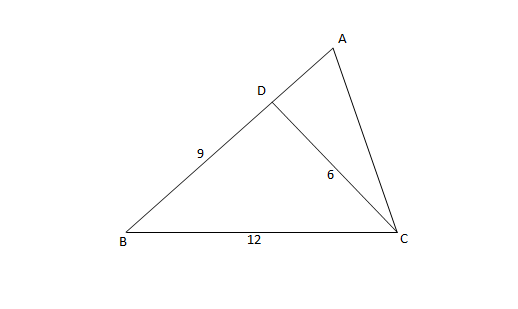Question 23

# Consider the triangle ABC shown in the following figure where BC = 12 cm, DB = 9 cm, CD = 6 and $$\angle{BCD} = \angle{BAC}$$What is the ratio of the perimeter of the triangle ADC to that of the triangle BDC?Solution

Consider triangles ABC and BDC
Angle B is common in both triangles. Also $$\angle A = \angle C$$
Since 2 angles are equal, the third angles $$\angle{ACB} = \angle{BDC}$$
Hence, triangle BCA $$\sim$$ BDC (BCA and BDC are similar)
AC/DC = BC/BD = AB/BC
BC = 12 cm, DB = 9 cm, CD = 6 cm
=> AC = 12/9 * 6 = 8 cm
AB = 8/6 * 12 = 16 cm
So, AD = 16 - 9 = 7 cm
Perimeter of ADC = 7+8+6 = 21 cm
Perimeter of BCD = 27 cm
Ratio = 21/27 = 7/9